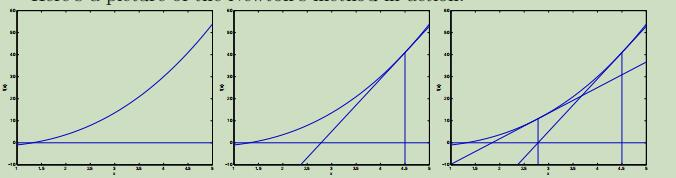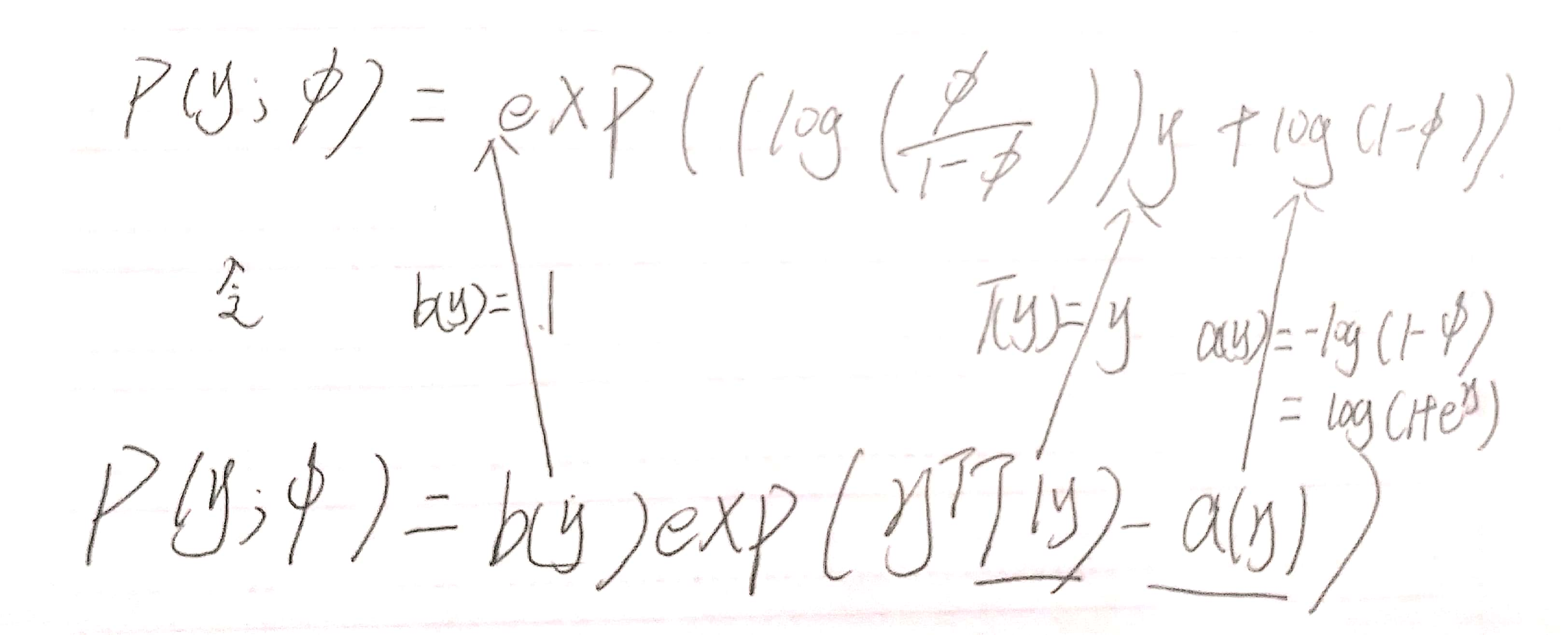# 吴恩达Stanford机器学习公开课（四）笔记

## Lecture 4 -Netwon's Method .Perceptron. Exponential Family. Generalized Linear Models.

Posted by Yunlongs on January 29, 2019

# Lecture 4 -Netwon’s Method .Perceptron. Exponential Family. Generalized Linear Models.

## Netwon’s Method

Netwon’s Method一种是不同于逻辑回归的算法，其目的仍是最大化$l(θ)$，但是在这里寻找的是另假设函数=0的点。下面介绍其算法思想： 如下面三幅图所示，首先确定一个点$θ_0$，之后在其对应函数点$f(θ_0)$做切线，记其切线与y=0的交点为$θ_1$，并进行迭代。# 广义线性模型（GLM,Generalized Linear Models）

## 指数族（The exponential family）在这里呢，我们是将自然参数为，η = log(φ/(1 − φ)).但如果用η来表示φ，φ =1/(1 + e^−η),这就是我们在逻辑回归中所用的sigmoid函数！

## 构造GLMS(Generalized Linear Models.)

问题描述：



1. y | x; θ ∼ ExponentialFamily(η).
2. h(x) = E[y|x].
3. 自然参数η和输入x线性相关：η = θTx.（如果η是向量，那么 ηi = θiTx.）


## Softmax Regression

φ1,..., φk−1 定义为产生每种结果的概率，φi = p(y = i; φ)且 φk = 1 −φ（1至k）。



$T(y)$和$y$的关系也可以表示为：$T(y)_i = 1\lbrace y = i\rbrace$ 还可以获得$E[T(y)_i] = P(y=i) = \phi _i$